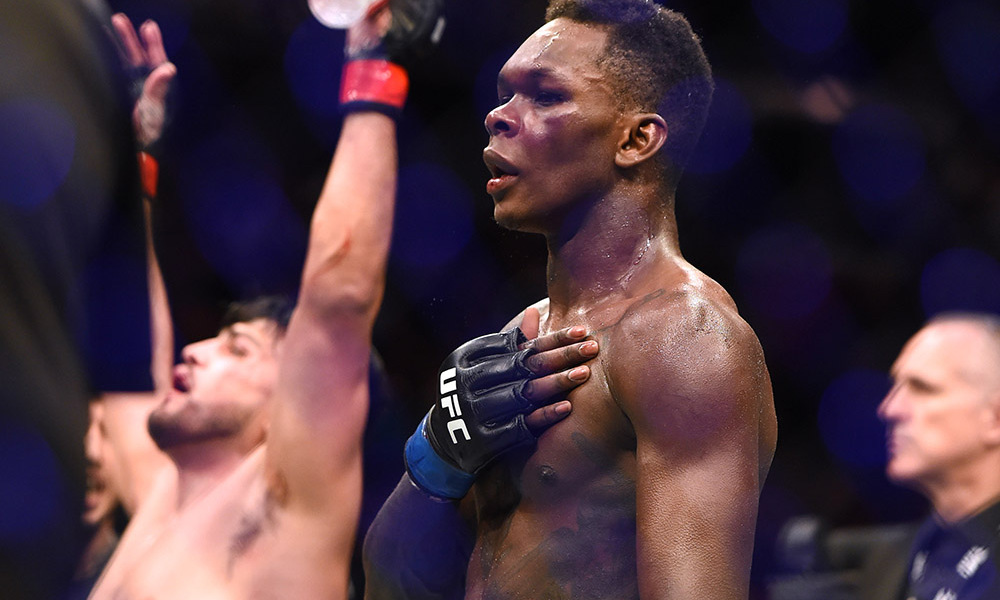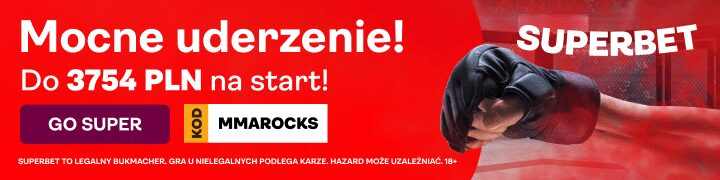Wypłaty po UFC 236:

Dustin Poirier (\$250,000 (bez bonusu za wygraną) = \$250,000) pok. Max Holloway (\$350,000)
Israel Adesanya (\$350,000 + (bez bonusu za wygraną) = \$350,000) pok. Kelvin Gastelum (\$150,000)
Khalil Rountree (\$35,000 + \$35,000 = \$70,000) pok. Eryk Anders (\$50,000)
Dwight Grant (\$12,000 + \$12,000 = \$24,000) pok. Alan Jouban (\$43,000)
Nikita Krylov (\$80,000 + \$80,000 = \$160,000) pok. Ovince Saint Preux (\$86,000)

Matt Frevola (\$10,000 + \$10,000 = \$20,000) pok. Jalin Turner (\$12,000)
Alexandre Pantoja (\$18,000 + \$18,000 = \$36,000) pok. Wilson Reis (\$34,000)
Max Griffin (\$20,000 + \$20,000 = \$40,000) pok. Zelim Imadaev (\$10,000)
Khalid Taha (\$10,000 + \$10,000 = \$20,000) pok. Boston Salmon (\$10,000)

Belal Muhammad (\$35,000 + \$35,000 = \$70,000) pok. Curtis Millender (\$31,000)
Montel Jackson (\$12,000 + \$12,000 = \$24,000) pok. Andre Soukhamthath (\$22,000)
Poliana Botelho (\$25,000 + \$25,000 = \$50,000) pok. Lauren Mueller (\$12,000)
Brandon Davis (\$18,000 + \$18,000 = \$36,000) pok. Randy Costa (\$12,000)#### 1 KOMENTARZ

1.bartolllox23

Krylov ile siana…

Witryna wykorzystuje Akismet, aby ograniczyć spam. Dowiedz się więcej jak przetwarzane są dane komentarzy.